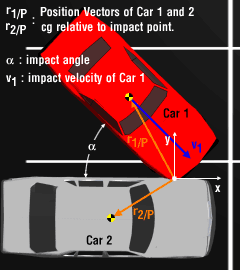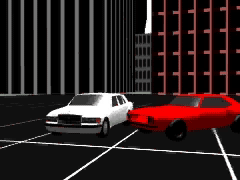Ch 8. Rigid Body Momentum/Impulse Multimedia Engineering Dynamics Impulse Momentum Eccentric Impact
 Chapter - Particle - 1. General Motion 2. Force & Accel. 3. Energy 4. Momentum - Rigid Body - 5. General Motion 6. Force & Accel. 7. Energy 8. Momentum 9. 3-D Motion 10. Vibrations Appendix Basic Math Units Basic Equations Sections Search eBooks Dynamics Fluids Math Mechanics Statics Thermodynamics Author(s): Kurt Gramoll ©Kurt GramollDYNAMICS - CASE STUDY IntroductionProblem Diagram After a particularly long lunch, an employee, in a hurry to return to work, slams his car (Car 1) into his boss's new Mercedes (Car 2), resulting in immediate termination of his employment. What is known: Car 1 weight w1 = 2,500 lb Car 2 weight w2 = 3,500 lb Car 1 moment of inertia about the CG    I1-cg = 1,164.6 slug-ft2 Car 2 moment of inertia    I2-cg = 2,101.5 slug-ft2 Car 1 velocity v1 = 52 mph Car 2 velocity v2 = 0 mph impact angle α = 45° position vectors for Cars' CGs r1/P = (3sinα - 6cosα)i + (6sinα + 3cosα)j ft r2/P = -5i - 3j ft coefficient of restitution e = 0.2Problem Graphic Question What are the translational and rotational velocities of the two vehicles immediately after the impact? Assume the bodies are perfectly rigid and the impact is elastic. Approach Use the Conservation of Angular and Linear Momentum as well as the coefficient of restitution to determine the velocities of each car. Ignore the friction between the car and the ground.

Practice Homework and Test problems now available in the 'Eng Dynamics' mobile app
Includes over 400 problems with complete detailed solutions.
Available now at the Google Play Store and Apple App Store.